#### Chapter 12 Heron's Formula R.D. Sharma Solutions for Class 9th Exercise 12.1

Exercise 12.1

Q1. Find the area of triangles whose sides are respectively 150cm, 120cm and 200cm.

Solution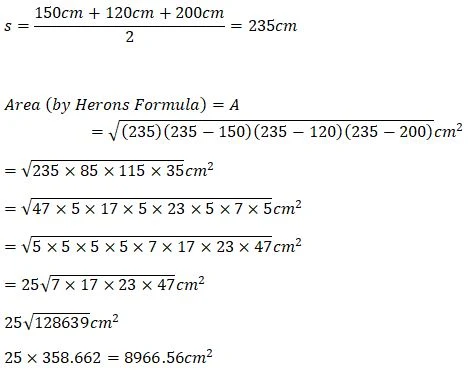Q2. Find the area of triangle whose sides are 9cm, 12cm and 15cm.

Solution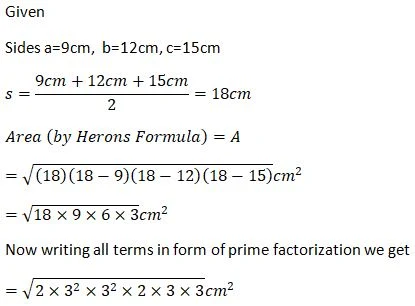Q3. Find the area of triangle two sides of which are 18cm and 10cm and perimeter is 42cm.

SolutionQ4. In a ∆ABC, AB=15cm, BC=13cm, and AC=14cm. Find the area of ∆ABC and hence its altitude on AC.

Solution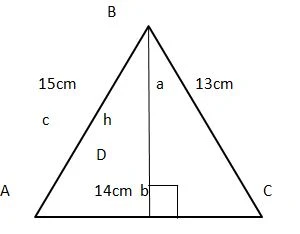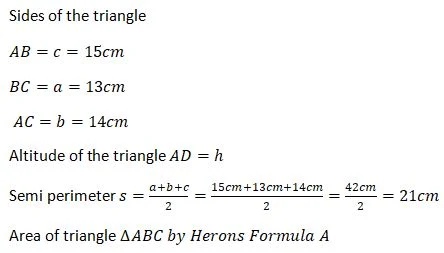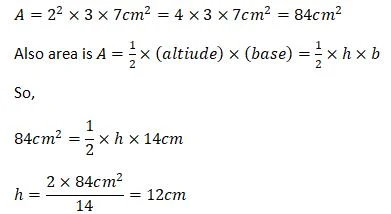Q5. The perimeter of triangular field is 540m and its sides are in ratio 25:17:12. Find the area of the triangle.

Solution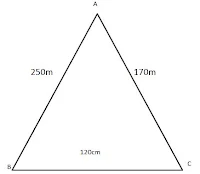Q6. The perimeter of the triangle is 300m. if its sides are in ratio 3:5:7. Find area of triangle.

Solution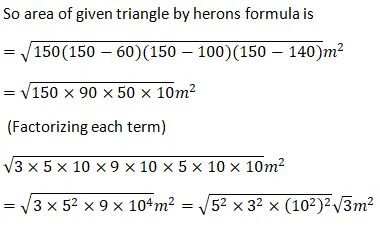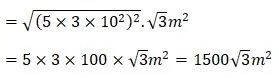Q7. The perimeter of triangular field is 240dm. If two of its sides are 78dm and 50dm, find the length of the perpendicular on the side 50dm from the opposite vertex.

Solution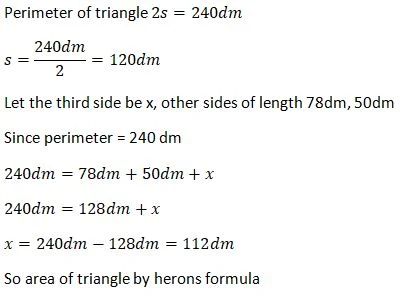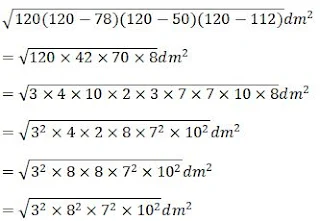Q8. A triangle has side 35cm, 54cm and 61cm. Find its area. Also, find its area. Find the smallest of the altitude.

Solution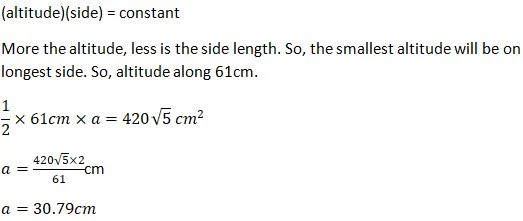Q9. The length of the sides of triangle are in ratio 3:4:5 and perimeter is 144cm. Find the area of the  triangle and the height corresponding to the longest side.

Solution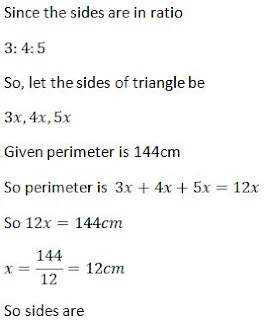Q10. The perimeter of the isosceles triangle is 42cm, and its base (3/2) times each of the equal sides. Find length of the each side of the triangle.

SolutionQ11. Find the area of shaded region in fig 12.12.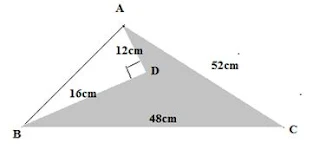Solution